# Project Appraisal and Risk

## Project Appraisal and Risk

### Project Appraisal and Risk

Chapter 6 Project Appraisal and Risk

1.         Objectives

1.1       Distinguish between risk and uncertainty in investment appraisal.
1.2       Define sensitivity analysis and discuss its usefulness in assisting investment decisions.
1.3       Apply sensitivity analysis to investment projects and explain the meaning of the findings.
1.4       Define and apply an expected value (EV) and discuss the usefulness of probability analysis in assisting investment decisions.
1.5       Discuss the use of simulation to take account of risk and uncertainty in investment appraisal.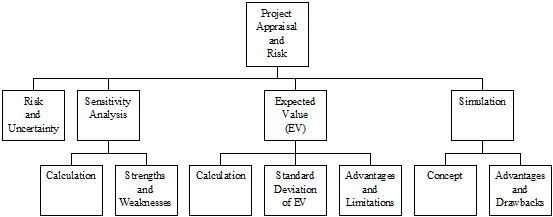2.         Risk and Uncertainty

2.1       Investment appraisal faces the following problems:
(a)        all decisions are based on forecasts;
(b)        all forecasts are subject to uncertainty;
(c)        this uncertainty needs to be reflected in the financial evaluation.
2.2       The decision maker must distinguish between:
(a)        Risk – can be quantifiable and be applied to a situation where there are several possible outcomes and, on the basis of past relevant experience, probabilities can be assigned to the various outcomes that could prevail.
(b)        Uncertainty – is unquantifiable and can be applied to a situation where there are several possible outcomes but there is little past experience to enable the probability of the possible outcomes to be predicted.
2.3       In investment appraisal the areas of concern are therefore the accuracy of the estimates concerning:
(a)        Project life
(b)        Predicted cash flows and associated probabilities
(c)        Discount rate used

3.         Sensitivity Analysis

3.1       Sensitivity analysis typically involves posing “what if?” questions. For example, what if demand fell by 10% compared to our original forecasts? Would the project still be viable?
3.2       In project appraisal, sensitivity analysis assesses how responsive the project’s NPV is to changes in the variables used to calculate that NPV.
3.3       The NPV could depend on a number of uncertain independent variables.
(a)        Selling price
(b)        Sales volume
(c)        Cost of capital
(d)        Initial cost
(e)        Operating costs
(f)        Benefits
3.4       Sensitivity analysis therefore provides an indication of why a project might fail. Management should review critical variables to assess whether or not there is a strong possibility of events occurring which will lead to a negative NPV. Management should also pay particular attention to controlling those variables to which the NPV is particularly sensitive, once the decision has been taken to accept the investment.
3.5       A simple approach to deciding which variables the NPV is particularly sensitive to is to calculate the sensitivity of each variable:

 Sensitivity = NPV % PV of project variable

3.6       The lower the percentage, the more sensitive is NPV to that project variable as the variable would need to change by a smaller amount to make the project non-viable.

3.7

EXAMPLE 1

ABC Co is considering a project with the following cash flows.

 Year Initial investment (\$000) Variable costs (\$000) Cash inflows (\$000) Net cash flows (\$000) 0 7,000 1 (2,000) 6,500 4,500 2 (2,000) 6,500 4,500

Cash flows arise from selling 650,000 units at \$10 per unit. ABC Co has a cost of capital of 8%.

Required:

Measure the sensitivity of the project to changes in variables.

Solution:

The PVs of the cash flow are as follows.

 Year Discount factor 8% PV of initial investment PV of variable costs PV of cash inflows PV of net cash flow \$000 \$000 \$000 \$000 0 1.000 (7,000) (7,000) 1 0.926 (1,852) 6,019 4,167 2 0.857 (1,714) 5,571 3,857 (7,000) (3,566) 11,590 1,024

NPV = 1,024

The project has a positive NPV and would appear to be worthwhile. The sensitivity of each project variable is as follows.

(a)      Initial investment
Sensitivity = 1,024 / 7,000 x 100% = 14.6%

(b)      Sales volume
Sensitivity = 1,024 / (11,590 – 3,566) x 100% = 12.8%

(c)      Selling price
Sensitivity = 1,024 / 11,590 x 100% = 8.8%

(d)      Variable costs
Sensitivity = 1,024 / 3,566 x 100% = 28.7%

(e)      Cost of capital. We need to calculate the IRR of the project.

 Year Net cash flow Discount factor 15% PV Discount factor 20% PV \$000 \$000 \$000 0 (7,000) 1 (7,000) 1 (7,000) 1 4,500 0.870 3,915 0.833 3,749 2 4,500 0.756 3,402 0.694 3,123 317 (128)

IRR = 0.15 +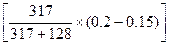= 18.56%
The cost of capital can therefore increase by 133% before the NPV becomes negative.

The elements to which the NPV appears to be most sensitive are the selling price followed by the sales volume. Management should thus pay particular attention to these factors so that they can be carefully monitored.

3.8       Strengths and weaknesses of sensitivity analysis

 Strengths Weaknesses (a)     No complicated theory to understand. (b)     Information will be presented to management in a form which facilitates subjective judgement to decide the likelihood of the various possible outcomes considered. (c)     Identifies areas which are crucial to the success of the project. If the project is chosen, those areas can be carefully monitored. (d)     Indicates just how critical are some of the forecasts which are considered to be uncertain. (a)      It assumes that changes to variables can be made independently, e.g. material prices will change independently of other variables. This is unlikely. If material prices went up the firm would probably increase selling price at the same time and there would be little effect on NPV. A technique called simulation (see later) allows us to change more than one variable at a time. (b)     It only identifies how far a variable needs to change. It does not look at the probability of such a change. In the above analysis, sales volume appears to be the most crucial variable, but if the firm were facing volatile raw material markets a 65% change in raw material prices would be far more likely than a 29% change in sales volume. (c)      It is not an optimising technique. It provides information on the basis of which decisions can be made. It does not point directly to the correct decision.

4.         Expected Value (EV)

4.1       When there are a number of possible outcomes for a decision and probabilities can be assigned to each, then an EV may be calculated.

 4.2 CONCEPT The EV is the weighted average of all possible outcomes, with the weightings based on the probability estimates. The formula for calculating an EV is: EV =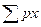Where: p = the probability of an outcome x = the value of an outcome

4.3

EXAMPLE 2

A firm has to choose between three mutually exclusive, the outcomes of which depend on the state of the economy. The following estimates have been made:

 State of the economy Recession Stable Growing Probability 0.5 0.4 0.1 NPV (\$000) NPV (\$000) NPV (\$000) Project A 100 200 1,400 Project B 0 500 600 Project C 180 190 200

Determine which project should be selected on the basis of expected market values.

Solution:

Project A

 State of the economy Probability Project NPV EV \$000 \$000 Recession 0.5 100 50 Stable 0.4 200 80 Growing 0.1 1,400 140 270

Project B

 State of the economy Probability Project NPV EV \$000 \$000 Recession 0.5 0 0 Stable 0.4 500 200 Growing 0.1 600 60 260

Project C

 State of the economy Probability Project NPV EV \$000 \$000 Recession 0.5 180 90 Stable 0.4 190 76 Growing 0.1 200 20 186

On the basis of expected values, Project A should be selected.
However, it should be noted that Project A is also the most risky option as it has the widest range of potential outcomes.

4.4

EXAMPLE 3

ABC Co is considering an investment of \$460,000 in a non-current asset expected to generate substantial cash inflows over the next five years. Unfortunately the annual cash flows from this investment are uncertain, but the following probability distribution has been established:

 Annual cash flow (\$) Probability 50,000 0.3 100,000 0.5 150,000 0.2

At the end of its five-year life, the asset is expected to sell for \$40,000. The cost of capital is 5%.

Should the investment be undertaken?

Solution:

Expected annual cash flows are:

 Annual cash flow Probability EV 50,000 0.3 15,000 100,000 0.5 50,000 150,000 0.2 30,000 95,000

NPV calculation:

 Time Cash flow DF 5% PV 0 (460,000) 1.000 (460,000) 1 95,000 4.329 411,255 2 40,000 0.784 31,360 NPV = (17,385)

As the ENPV is negative, the project should not be undertaken.

An alternative approach would be to calculate three separate NPVs and then combine them, giving the following figures:

 Annual cash flow Probability PV 50,000 0.3 (212,190) 100,000 0.5 4,260 150,000 0.2 220,710

ENPV = 0.3 x (212,190) + 0.5 x 4,260 + 0.2 x 220,710 = (17,385)

Even though the ENPV is negative these figures show that there is a 70% chance of the project giving a positive NPV. Some investors may consider the project acceptable on this basis.

4.5

A company is considering whether to invest in equipment for providing a new service to its clients. The equipment will cost \$100,000 and will have a disposal value of \$20,000 after four years. Estimates of sales and incremental fixed cost cash expenditures are as follows.

 Annual cash flow (\$) Probability 600,000 0.4 700,000 0.4 800,000 0.2

The company expects to achieve a contribution/sales ratio of 40% on all the services it provides. Incremental fixed costs will be \$215,000 per annum. The project has a four-year life.

The company’s cost of capital is 9%.

Calculate the three possible NPVs an the expected NPV. Comment on your results.

4.6

EXAMPLE 4

A company is considering a project involving the outlay of \$300,000 which it estimates will generate cash flows over its two year life at the probabilities shown in the following table.
Year 1

 Annual cash flow (\$) Probability 100,000 0.25 200,000 0.50 300,000 0.25

Year 2

 If cash flows in Year 1 is: There is probability of: That the cash flow in Year 2 will be: 100,000 0.25 Nil 0.50 100,000 0.25 200,000 200,000 0.25 100,000 0.50 200,000 0.25 300,000 300,000 0.25 200,000 0.50 300,000 0.25 350,000

The company’s investment criterion for this type of project is 10% DCF.

You are required to calculate the expected value of the project’s NPV and the probability that the NPV will be negative.

Solution:

Calculate expected value of the NPV.
First we need to draw up a probability distribution of the expected cash flows. We begin by calculating the PV of the cash flows.

 Year Cash flow DF 10% PV \$000 \$000 1 100 0.909 90.9 1 200 0.909 181.8 1 300 0.909 272.7 2 100 0.826 82.6 2 200 0.826 165.2 2 300 0.826 247.8 2 350 0.826 289.1
 Year 1 PV of cash flow Prob. Year 2 PV of cash flow Prob. Joint Prob. Total PV of cash inflows EV of PV of cash inflows \$000 \$000 \$000 \$000 (a) (b) (c) (d) (b) x (d) (a) + (c) 90.9 0.25 0.0 0.25 0.0625 90.9 5.681 90.9 0.25 82.6 0.50 0.125 173.5 21.688 90.0 0.25 165.2 0.25 0.0625 256.1 16.006 181.8 0.50 82.6 0.25 0.125 264.4 33.05 181.8 0.50 165.2 0.50 0.250 347 86.75 181.8 0.50 247.8 0.25 0.125 429.6 53.70 272.7 0.25 165.2 0.25 0.0625 437.9 27.369 272.7 0.25 247.8 0.50 0.125 520.5 65.063 272.7 0.25 289.1 0.25 0.0625 561.8 35.113 344.420
 \$ EV of PV of cash inflows 344,420 Less: Project cost 300,000 EV of NPV 44,420

Measure risk.
Since the EV of the NPV is positive, the project should go ahead unless the risk is unacceptably high. The probability that the project will have a negative NPV is the probability that the total PV of cash inflows is less than \$300,000. From the column headed ‘Total PV of cash inflows’, we can establish that this probability is 0.0625 + 0.125 + 0.0625 + 0.125 = 0.375 or 37.5%. This might be considered an unacceptably high risk.

(A)       The standard deviation of the NPV

4.7       The disadvantage of using the EV of NPV approach to assess the risk of the project is that the construction of the probability distribution can become very complicated. If we were considering a project over 4 years, each year have five different forecasted cash flows, there would be 625 (54) NPVs to calculate. To avoid all of these calculations, an indication of the risk may be obtained by calculating the standard deviation of the NPV.

4.8

EXAMPLE 5

Frame Co is considering which of two mutually exclusive projects, A or B, to undertake. There is some uncertainty about the running costs with each project, and a probability distribution of the NPV for each project has been estimated, as follows.

 Project A Probability Project B Probability NPV NPV \$000 \$000 – 20 0.15 + 5 0.2 + 10 0.20 + 15 0.3 + 20 0.35 + 20 0.4 + 40 0.30 + 25 0.1

You are required to decide which project should the company choose, if either.

Solution:

We can begin by calculating the EV of the NPV for each project.

 Project A Project B NPV Prob. EV NPV Prob. EV \$000 \$000 \$000 \$000 – 20 0.15 (3.0) 5 0.2 1.0 10 0.20 2.0 15 0.3 4.5 20 0.35 7.0 20 0.4 8.0 40 0.30 12.0 25 0.1 2.5 18.0 16.0

Project A has a higher EV of NPV, but what about the risk of variation in the NPV above or below the EV? This can be measured by the standard deviation of the NPV.

The standard deviation of a project’s NPVs, can be calculated as: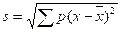, where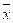is the EV of the NPV.

 Project A,= 18 Project B,= 16 x p x -p(x -)2 x p x -p(x -)2 – 20 0.15 – 38 216.6 5 0.2 – 11 24.2 10 0.20 – 8 12.8 15 0.3 – 1 0.3 20 0.35 + 2 1.4 20 0.4 + 4 6.4 40 0.30 + 22 145.2 25 0.1 + 9 8.1 376.0 39.0
 Project A Project B Standard deviation s =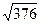= 19.391 = \$19,391 Standard deviation s =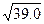= 6.245 = \$6,245

Although Project A has a higher EV of NPV, it also has a higher standard deviation of NPV, and so has greater risk associated with it.

Which project should be selected? Clearly it depends on the attitude of the company’s management to risk.
(a)      If management are prepared to take the risk of a low NPV in the hope of a high NPV they will opt for project A.
(b)      If management are risk-averse, they will opt for the less risky project B.

4.9       Advantages and limitation of expected values

 Advantages Limitations (a)     The technique recognises that there are several possible outcomes and is, therefore, more sophisticated than single value forecasts. (b)     Enables the probability of the different outcomes to be quantified. (c)     Leads directly to a simple optimising decision rule. (d)     Calculations are relatively simple. (a)      By asking for a series of forecasts the whole forecasting procedure is complicated. Inaccurate forecasting is already a major weakness in project evaluation. The probabilities used are also usually very subjective. (b)     The EV is merely a weighted average of the probability distribution, indicating the average payoff if the project is repeated many times. (c)      The EV gives no indication of the dispersion of possible outcomes about the EV. The more widely spread out the possible results are, the more risky the investment is usually seen to be. The EV ignores this aspect of the probability distribution. (d)     In ignoring risk, the EV technique also ignores the investor’s attitude to risk. Some investors are more likely to take risks than others.

5.         Simulation (模擬)

5.1       Sensitivity analysis considered the effect of changing one variable at a time. Simulation improves on this by looking at the impact of many variables changing at the same time.
5.2       Using mathematical, it produces a distribution of the possible outcomes from the project. The probability of different outcomes can then be calculated.

5.3

EXAMPLE 6

The following probability estimates have been prepared for a proposed project.

 Year Probability Cost of equipment 0 1.00 (40,000) Revenue each year 1 – 5 0.15 40,000 0.40 50,000 0.30 55,000 0.15 60,000 Running costs each year 1 – 5 0.10 25,000 0.25 30,000 0.35 35,000 0.30 40,000

The cost of capital is 12%. Assess how a simulation model might be used to assess the project’s NPV.

Solution:

A simulation model could be constructed by assigning a range of random number digits to each possible value for each of the uncertain variables. The random numbers must exactly match their respective probabilities. This is achieved by working upwards cumulatively from the lowest to the highest cash flow values and assigning numbers that will correspond to probability groupings as follows.

 Revenue Running costs \$ Prob. Random No. \$ Prob. Random No. 40,000 0.15 00 – 14 * 25,000 0.10 00 – 09 50,000 0.40 15 – 54 ** 30,000 0.25 10 – 34 55,000 0.30 55 – 84 *** 35,000 0.35 35 – 69 60,000 0.15 85 – 99 40,000 0.30 70 – 99

* Probability is 0.15 (15%). Random numbers are 15% of range 00 – 99.
** Probability is 0.40 (40%). Random numbers are 40% of range 00 – 99 but starting at 15.
*** Probability is 0.30 (30%). Random numbers are 30% of range 00 – 99 but starting at 55.

For revenue, the selection of a random number in the range 00 and 14 has a probability of 0.15. This probability represents revenue of \$40,000. Numbers have been assigned to cash flows so that when numbers are selected at random, the cash flows have exactly the same probability of being selected as is indicated in their respective probability distribution above.

Random numbers would be generated, for example by a computer program, and these would be used to assign values to each of the uncertain variables.

For example, if random numbers 378420015689 were generated, the values assigned to the variables would be as follows.

 Revenue Running costs Calculation Random No. Value Random No. Value \$ \$ 1 37 50,000 84 40,000 2 20 50,000 01 25,000 3 56 55,000 89 40,000

A computer would calculate the NPV many times over using the values established in this way with more random numbers, and the results would be analysed to provide the following.

(a)      An expected value for the project.
(b)     A statistical distribution pattern for the possible variation in the NPV above or below this average.

The decision whether to go ahead with the project would then be made on the basis of expected return and risk.

5.4       Advantages and drawbacks of simulation

 Advantages Drawbacks (a)     It includes all possible outcomes in the decision-making process. (b)     It is a relatively easily understood technique. (c)     It has a wide variety of applications (inventory control, component replacement, etc.) (a)      Models can become extremely complex and the time and costs involved in their construction can be more than is gained from the improved decisions. (b)     Probability distributions may be difficult to formulate.

Examination Style Questions

Question 1 – NPV and Sensitivity Analysis
Betula Co has recently acquired a patent, at a cost of \$1·3 million, which would allow it to manufacture and sell a product for measuring an individual’s bio-rhythms. The marketing department of Betula Co estimates that 300,000 units of this product can be sold during each of the next four years. The selling price of the product is \$24 and variable costs are estimated to be \$18 per product. Fixed costs (excluding depreciation) are estimated to be \$2·2 million per year. This figure consists of \$1·4 million additional fixed costs and \$0·8 million existing fixed costs that are to be apportioned to the new product.

To manufacture the new product, equipment costing \$1·5 million will be acquired immediately. The estimated residual value of this equipment in four years’ time is \$0·5 million. The company calculates depreciation on a straight-line basis.

The cost of capital of Betula Co is 9%.

Betula is currently considering whether to go ahead with the project.

Required:

(a)     Calculate the net present value of the decision to go ahead with the project.
(5 marks)
(b)     Undertake sensitivity analysis to show the change needed to each of the following before a zero NPV is achieved:
(i)      discount rate;
(ii)     initial outlay on equipment;
(iii)    net annual operating cash flows;
(iv)    residual value of the equipment.
(11 marks)
(c)     Evaluate briefly the information produced in your answer to (a) and (b) above and state, with reasons, whether or not the project should go ahead.                                                         (4 marks)
(Total 20 marks)

Question 2 – Expected value and Working Capital Management
ZSE Co is concerned about exceeding its overdraft limit of \$2 million in the next two periods. It has been experiencing considerable volatility in cash flows in recent periods because of trading difficulties experienced by its customers, who have often settled their accounts after the agreed credit period of 60 days. ZSE has also experienced an increase in bad debts due to a small number of customers going into liquidation.

The company has prepared the following forecasts of net cash flows for the next two periods, together with their associated probabilities, in an attempt to anticipate liquidity and financing problems. These probabilities have been produced by a computer model which simulates a number of possible future economic scenarios. The computer model has been built with the aid of a firm of financial consultants.

 Period 1 cash flow Probability Period 2 cash flow Probability \$000 \$000 8,000 10% 7,000 30% 4,000 60% 3,000 50% (2,000) 30% (9,000) 20%

ZSE Co expects to be overdrawn at the start of period 1 by \$500,000.

Required:

(a)     Calculate the following values:
(i)      the expected value of the period 1 closing balance;
(ii)     the expected value of the period 2 closing balance;
(iii)    the probability of a negative cash balance at the end of period 2;
(iv)    the probability of exceeding the overdraft limit at the end of period 2.
Discuss whether the above analysis can assist the company in managing its cash flows. (13 marks)
(b)     Identify and discuss the factors to be considered in formulating a trade receivables management policy for ZSE Co.                                                                                                          (8 marks)
(c)     Discuss whether profitability or liquidity is the primary objective of working capital management.                                                                                                                              (4 marks)
(Total 25 marks)
(ACCA F9 Financial Management June 2010 Q1)

Source: https://hkiaatevening.yolasite.com/resources/FMNotes/Chapter6-AppraisalRisk.doc

Web site to visit: https://hkiaatevening.yolasite.com

Author of the text: indicated on the source document of the above text

If you are the author of the text above and you not agree to share your knowledge for teaching, research, scholarship (for fair use as indicated in the United States copyrigh low) please send us an e-mail and we will remove your text quickly. Fair use is a limitation and exception to the exclusive right granted by copyright law to the author of a creative work. In United States copyright law, fair use is a doctrine that permits limited use of copyrighted material without acquiring permission from the rights holders. Examples of fair use include commentary, search engines, criticism, news reporting, research, teaching, library archiving and scholarship. It provides for the legal, unlicensed citation or incorporation of copyrighted material in another author's work under a four-factor balancing test. (source: http://en.wikipedia.org/wiki/Fair_use)

The information of medicine and health contained in the site are of a general nature and purpose which is purely informative and for this reason may not replace in any case, the council of a doctor or a qualified entity legally to the profession.

#### Project Appraisal and Risk

The texts are the property of their respective authors and we thank them for giving us the opportunity to share for free to students, teachers and users of the Web their texts will used only for illustrative educational and scientific purposes only.

All the information in our site are given for nonprofit educational purposes

##### Project Appraisal and Risk

Topics and Home
Contacts
Term of use, cookies e privacy